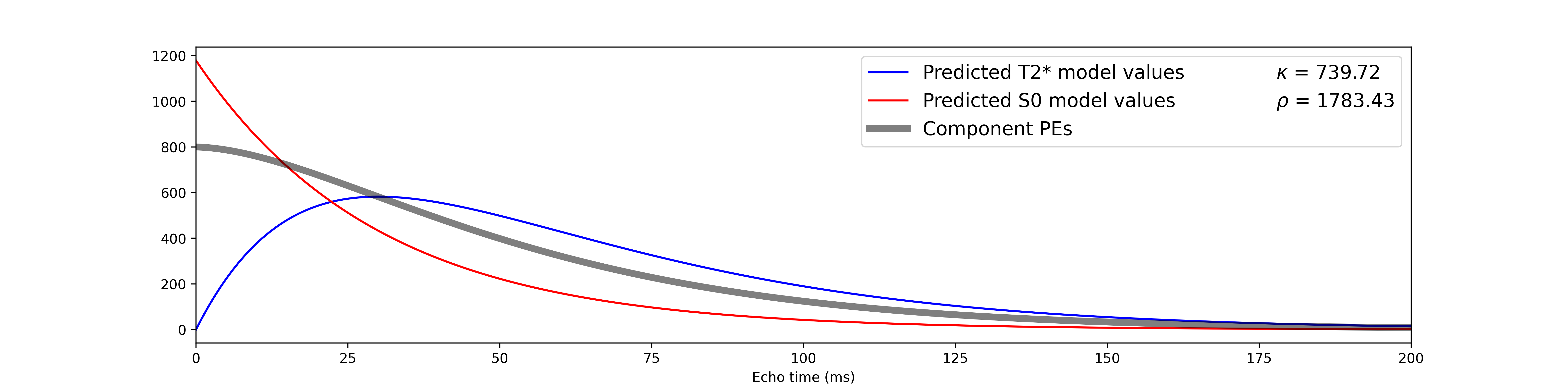# TE (In)Dependence Models

Functional MRI signal can be described in terms of fluctuations in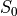and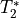. In the below equation,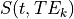is signal at a given timeand for a given echo time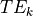.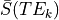is the mean signal across time for the echo time.is the difference inat timefrom the average.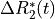is the difference in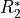at timefrom the average.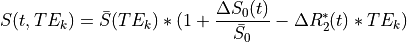If we ignore time, this can be simplified to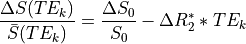In order to evaluate whether signal change is being driven by fluctuations in eitheror, one can break this overall model into submodels by zeroing out certain terms.

Important

Remember-is just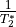For a TE-independence model, if there were no fluctuations in: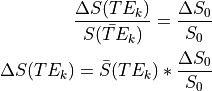Note that TE is not a parameter in this model. Hence, this model is TE-independent.

Also,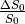is a scalar (i.e., doesn’t change with TE), so we can just ignore that, which means we only use(mean echo-wise signal).

Thus, the model becomes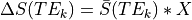, where we fit X to the data using regression and evaluate model fit.

For TEDPCA/TEDICA, we use regression to get parameter estimates (raw PEs; not standardized beta values) for component time-series against echo-specific data, and substitute those PEs for. Thus, to assess the TE-independence of a component, we use the model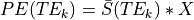, fit X to the data, and evaluate model fit.

For a TE-dependence model, if there were no fluctuations in: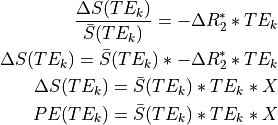Note that TE is a parameter in this model. Hence, it is TE-dependent.

## Applying our models to signal decay curves

As an example, let us simulate some data. We will simulate signal decay for two time points, as well as the signal decay for the hypothetical overall average over time. In one time point, onlywill fluctuate. In the other, onlywill fluctuate.

Caution

To make things easier, we’re simulating these data with echo times of 0 to 200 milliseconds, at 1ms intervals. In real life, you’ll generally only have 3-5 echoes to work with. Real signal from each echo will also be contaminated with random noise and will have influences from bothand.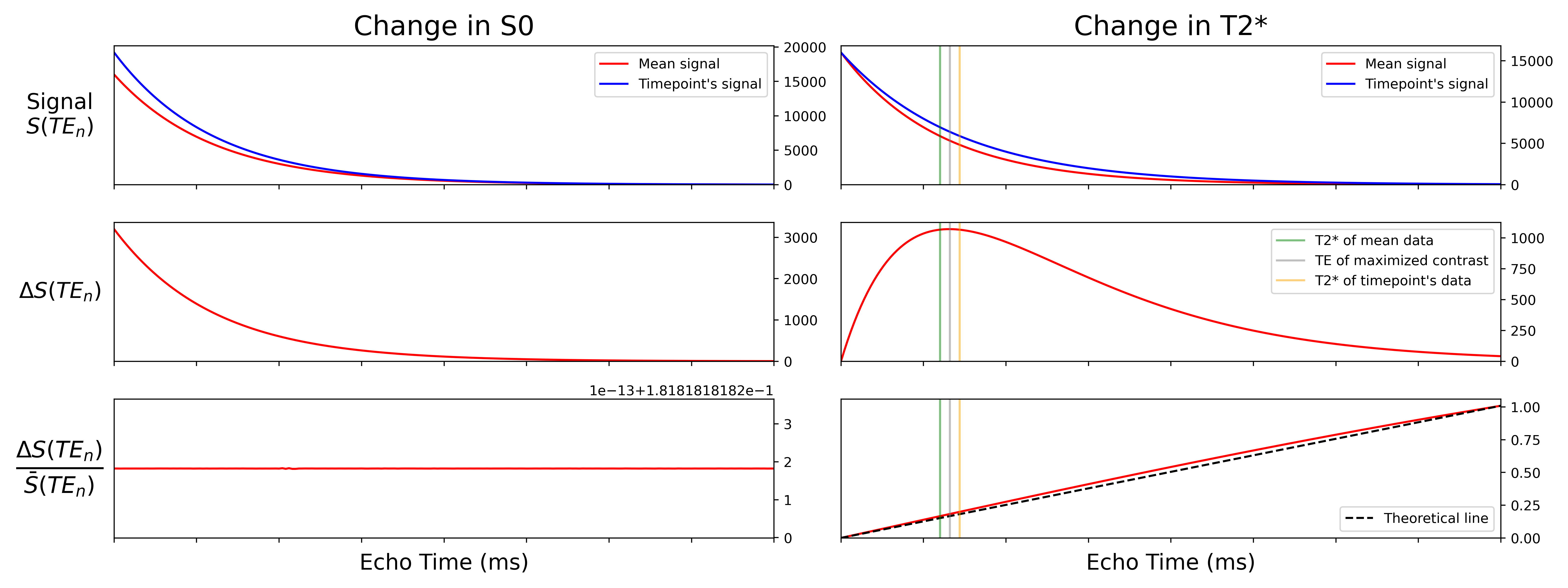We can see that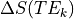has very different curves for the two simulated datasets. Moreover, as expected,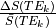is flat across echoes for the-fluctuating data and scales roughly linearly with TE for the-fluctuating data.

We then fit our TE-dependence and TE-independence models to thedata, which gives us predicted data for each model for each dataset.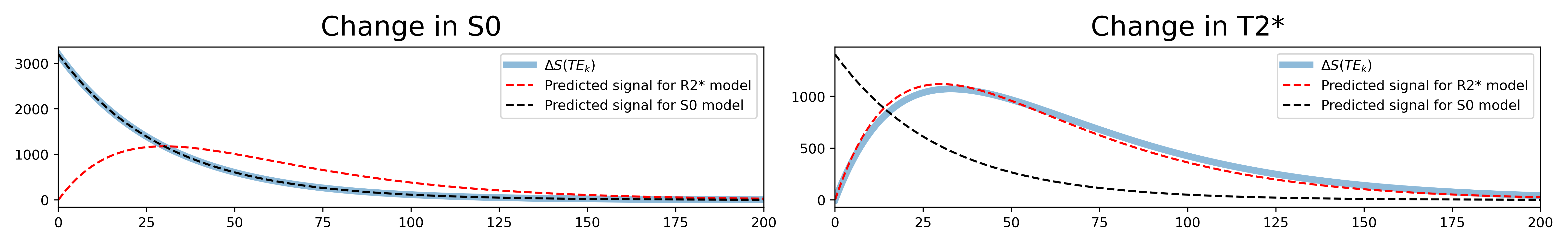As expected, themodel fits perfectly to the-fluctuating dataset, while themodel fits quite well to the-fluctuating dataset.

The actual model fits can be calculated as F-statistics. Then, the F-statistics per voxel are averaged across voxels into the Kappa and Rho pseudo-F-statistics.

## Applying our models to spatiotemporal decompositions

Now let us see how this extends to time series, components, and component parameter estimates.

We have the means to simulate- and-based fluctuations, so here we have generated two time series- one-based and one-based. Both time series share the same level of percent signal change (a standard deviation equivalent to 5% of the mean), although the mean(16000) is very different from the mean(30).

We can then average those two time series with different weights to create components that are- or-based to various degrees. In this case, bothandcontribute equally to the simulated time series. This simulated time series will act as our ICA component for this example.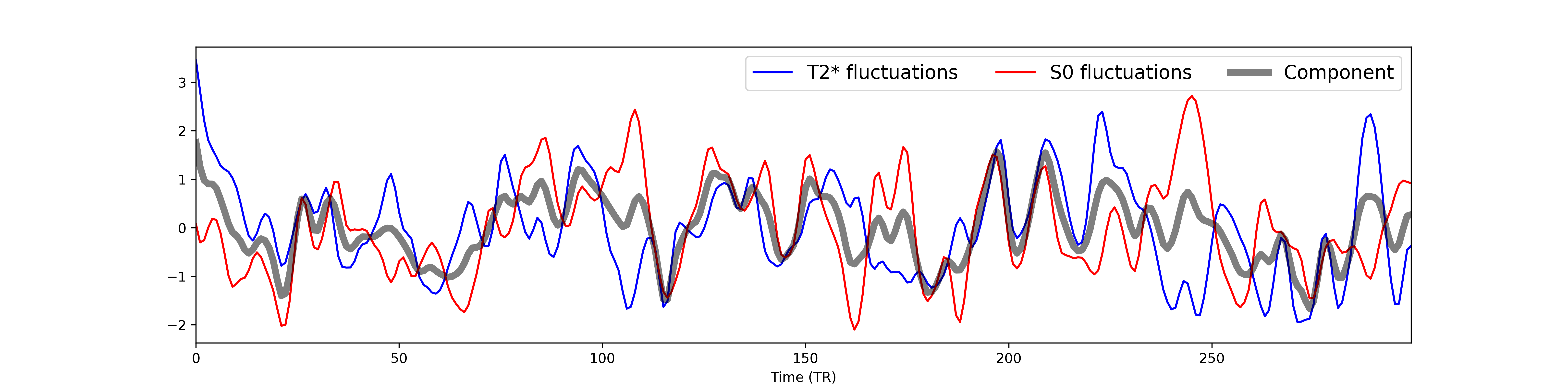We also simulate multi-echo data for a single voxel with the same levels ofandfluctuations as in the pureandtime series above. Here we show time series for a subset of echo times.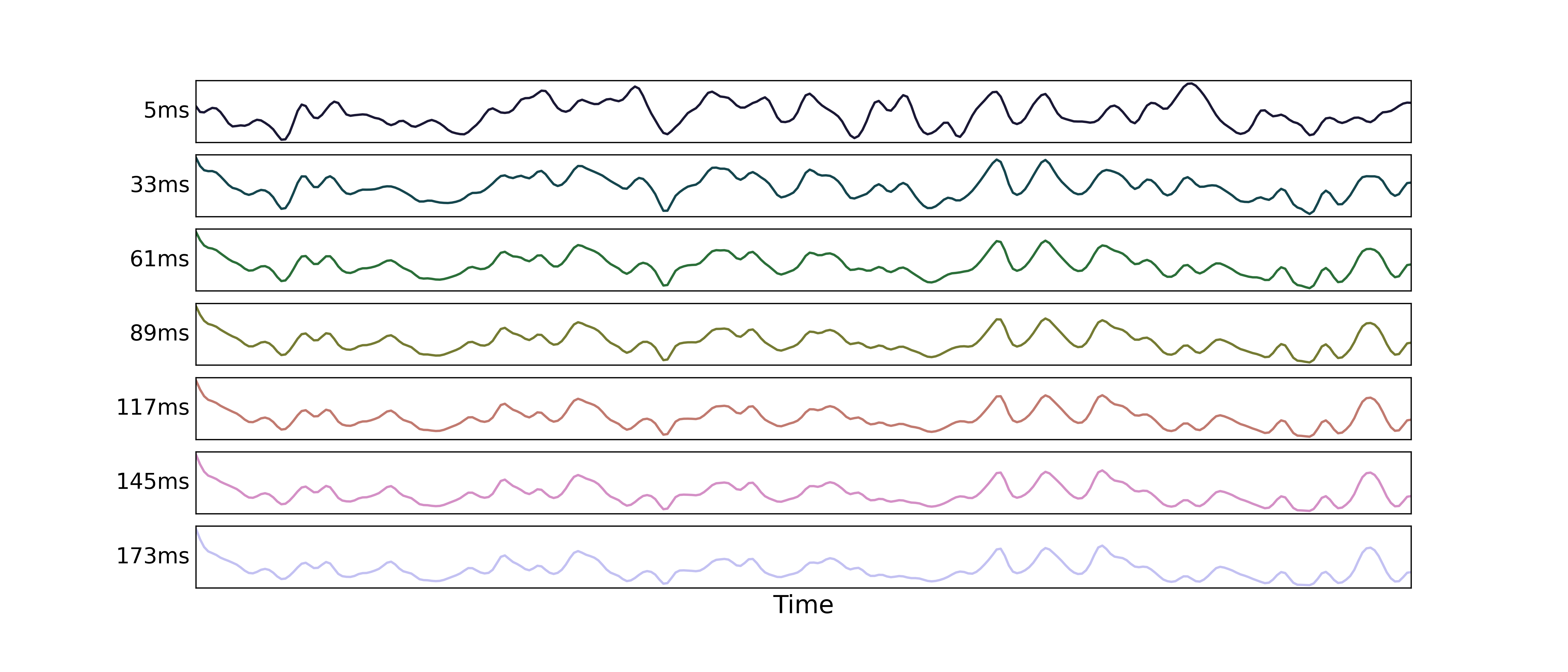And here we can see how those signals decay with echo time (again for only a subset of echo times):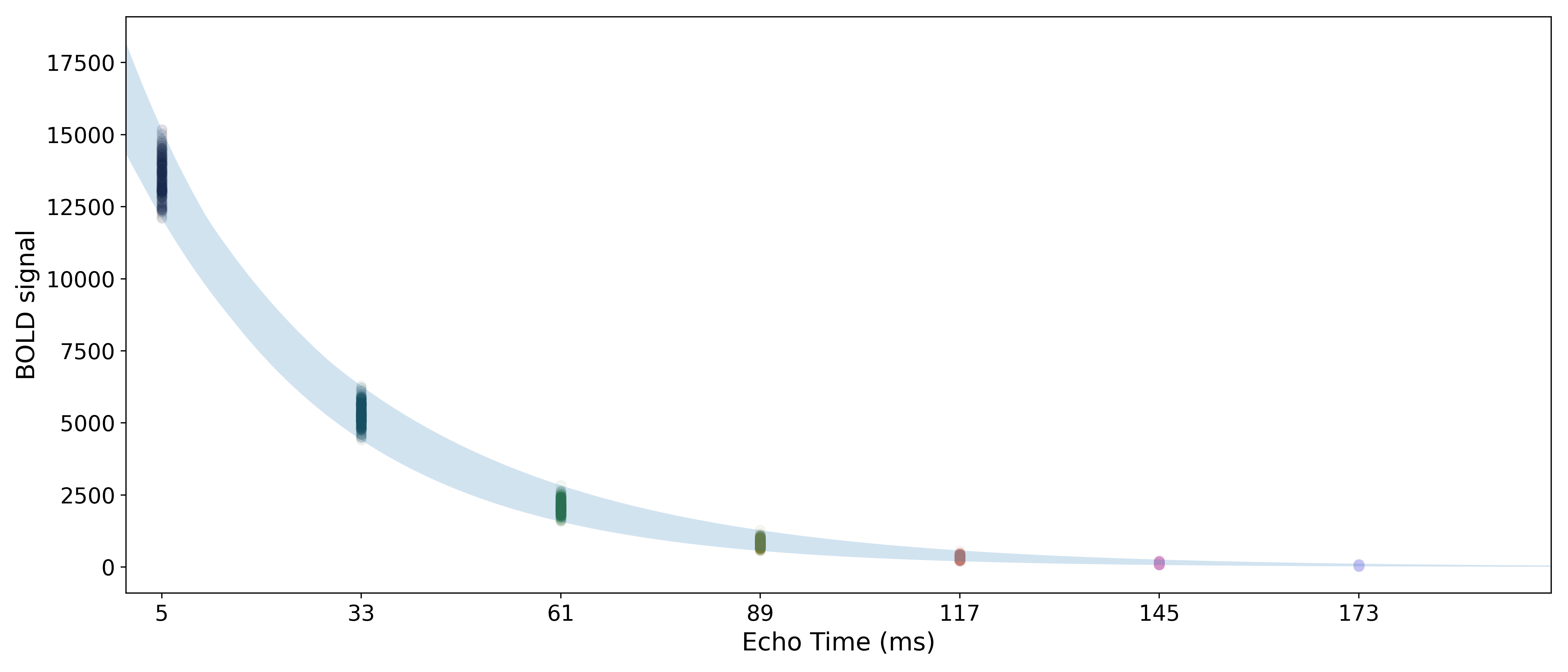We then run a regression for each echo’s data against the component time series, producing one parameter estimate for each echo time. The parameter estimates match the signal decay curve for, as seen above. We can thus apply the same TE-dependence and -independence models as above, in order to calculate single-voxel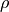and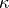values. Note that the metric values are extremely high, due to the inflated degrees of freedom resulting from using so many echoes in the simulations.

Attention

You may also notice that, despite the fact thatandfluctuate the same amount and that both contributed equally to the component,is much higher than.